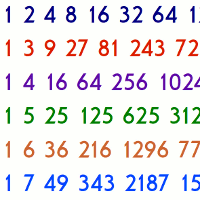# A Power Puzzle

### An idea for an exploratory math exercise

By

Here's an idea to get a class of kids exploring numbers with a calculator. At the end, I'll give a couple of challenges that should keep some occupied for a long time!

Let me introduce the puzzle by way of an example... Starting with the number 1, what happens when you keep multiplying by three? What digits does each of the numbers start with?

• Well, 1 starts with a 1. That's clear enough.
• Then, 3 starts with 3, and 9 starts with 9.
• Multiplying by 3 again, 27 starts with 2, then 81 starts with 8. And then, 243 starts with 2 again.
• What 'first digits' have we got so far? 1, 2, 3, 8 and 9.
• Multiplying 243 by 3 gives 729, so now we have 1, 2, 3, 7, 8 and 9.
• The next few powers of three are 2187, 6561, 19683 and 59049, so now we've got all possible starting digits except for 4!
• Going on, we get 177147, 531441, 1594323 and finally 4782969.
So the number 1 must be multiplied by 3 a total of 14 times before every possible 'first digit' is found.

The exercise is this - instead of multiplying by 3, what if you keep multiplying by other numbers, say 5? or 2.9? Or even pi?? Or what if you start with other numbers, such as 2, or 6.1?

Get the kids to try different combinations of starting number and multiplier, and record how many steps it takes for the starting digit to cover everything from 1 to 9.

Some important tips for doing this exercise, to make it more practical :

• Make sure the kids have calculators!
• The numbers on the calculators will get quite big sometimes - too big for a calculator. But the exercise can continue, if the child divides by some power of 10. In the example above, I could divide 177147 by 1000 to get 177.147, then keep going. Multiplying by 3 again and again gives 531.441, 1594.323 and then 4782.969. These all have the same 'first digits' as the 'real' products. So teach the kids to divide by 1000 every now and then when the calculator screen begins to get too full...!

• Start with 1, and keep multiplying by 2. Is the first digit ever seven??
• Can you find a multiplier and a starting number - that never covers all the digits? Or at least, not for a veeeeery looooong time? (When someone comes up with multipliers like 9.99999 or 1.00001, congratulate them and ask if they can find other kinds of 'long' multipliers!)
• Can you find the multiplier and starting number that takes as few steps as possible to cover every digit?
• If you want to get all the digits quickly, or slowly, is it more important to choose the starting number carefully, or the multiplier?

Well, that's all for now. I hope it helps!